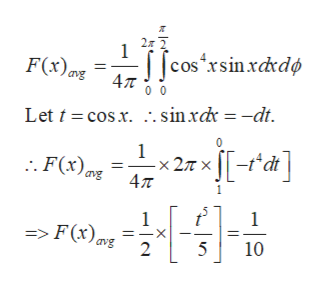# The radiation pattern of an antenna is given by F(x)= cos4 x with 0<x<pi/2.What will be the directivity of the antenna?

Question
18 views

The radiation pattern of an antenna is given by F(x)= cos4 x with 0<x<pi/2.

What will be the directivity of the antenna?

check_circle

Step 1

Directivity is a very important antenna parameter and it can be used to determine how concentrated the radiation is in a specific direction. If antenna radiation pattern F(x) is given, then the directivity can be expressed as

Step 2

Radiation pattern is expressed in spherical polar coordinates.

In this case, the radiation pattern is given as

Step 3

The maximum value of F(x) will be 1. The average value of F(x) can be computed in the following way:

...help_outlineImage Transcriptionclose||cos*xsin.xdrdø F(x)mz = ang Let t = cos.x. .. sinxdx = -dt. [[-*a] x 2.7 x [[-t°di] .. F(x)mg 4л avg => F(x) avg 10 fullscreen

### Want to see the full answer?

See Solution

#### Want to see this answer and more?

Solutions are written by subject experts who are available 24/7. Questions are typically answered within 1 hour.*

See Solution
*Response times may vary by subject and question.
Tagged in

### Electrical Engineering